BENDING MOMENT AND SHEARING FORCE DIAGRAMS 1

# 1 BENDING MOMENT AND SHEARING FORCE DIAGRAMS 1

## 1.1 Problem definition

Determinate the support pressures of a beam, which stresses by a line load.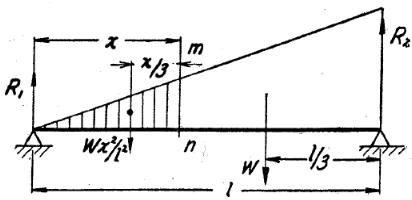Figure 1: Model by Timoshenko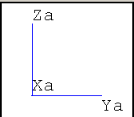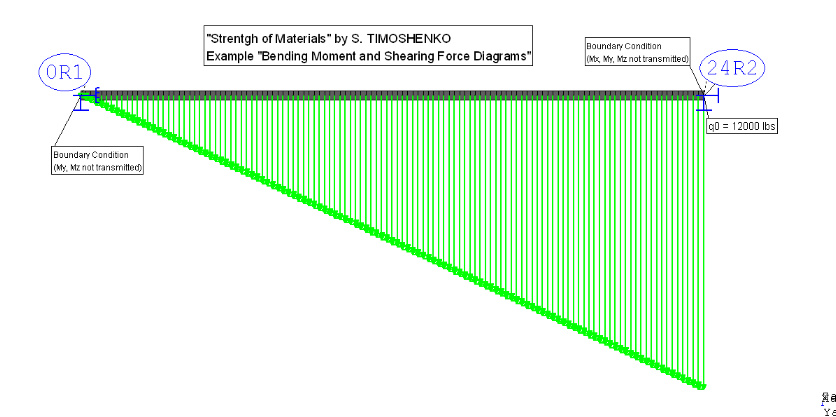Figure 2: Rohr2 Model

## 1.2 References (Timoshenko)

S. Timoshenko, Strength of Material, Part I, Elementary Theory and Problems, 3rd Edition, D. Van Nostrand Co., Inc., New York, NY, 1956, Chapter 3.22, pg. 85.

A profile of carbon steel stresses under an triangle- line load. The following parameters are given:

• Triangle-line Load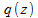• maximum of the line load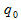• Length of the structural section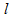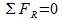Where: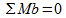Variable Description Unit Used Value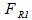Support load 0 R1 lbf 48000,00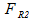Support load 24 R2 lbf 96000,00Total length ft 24,00Triangle-line load lbf 500,00Line load 24 R2 lbs/ ft 12000,00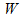Sum of support loads lbf 144000,00

Table 1: Overview of the used variables

## 1.3 Model description (ROHR2)

One 24 ft long structural section (I100) is used. A triangle-line load (500 lbs/ ft) is applied. The boundary conditions at both ends are: The left side of the beam, where the line load increases from zero is named 0R1 (My, Mz not transmitted) and the point of maximum stress is named 24R2 (Mx, My, Mz not transmitted). In ROHR2 it is necessary to break down the entire triangle-line load into many parts of constant loads. These loads have to be chosen so that the sum under the load surface is equal to the total line load. Seven different systems were generated for this model. It should illustrate, how the results (support loads) behave, when different discretization are used. In the Load case 1 the gravitational acceleration is not considered.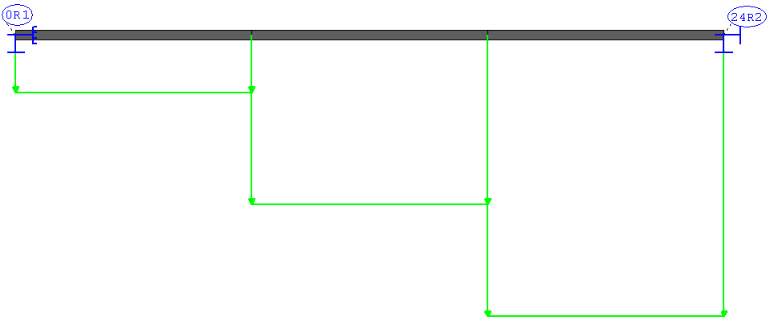Figure 4: 8 inch model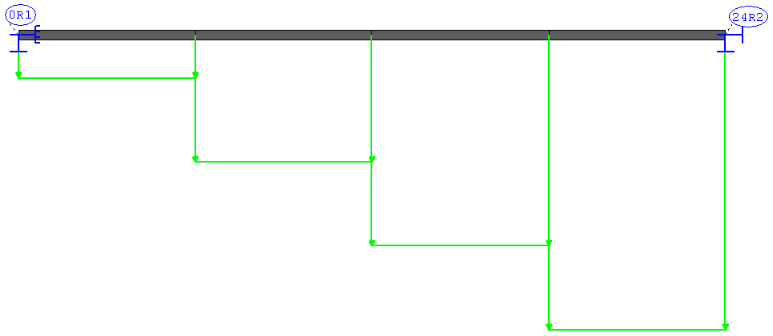Figure 3: 6 inch model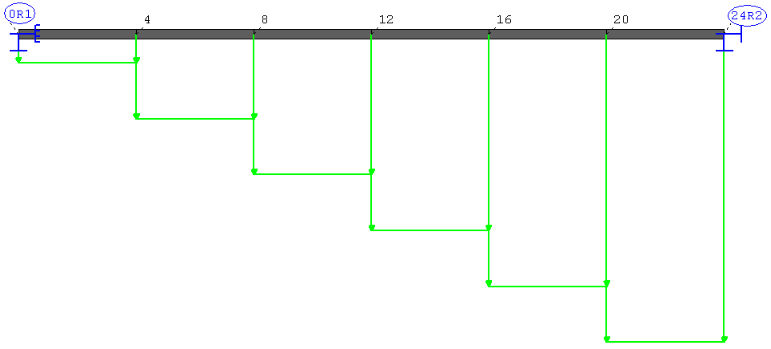Figure 6: 4 inch model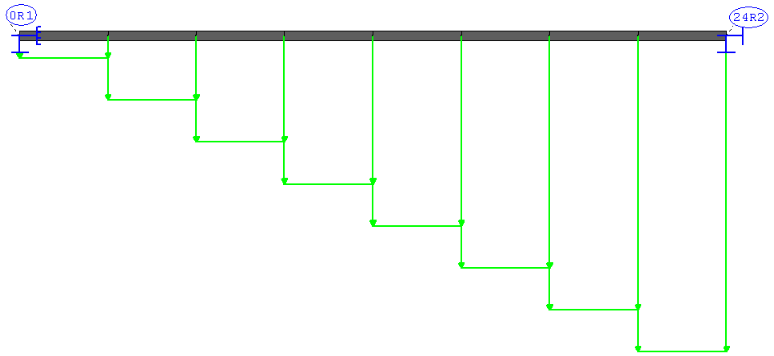Figure 5: 3 inch model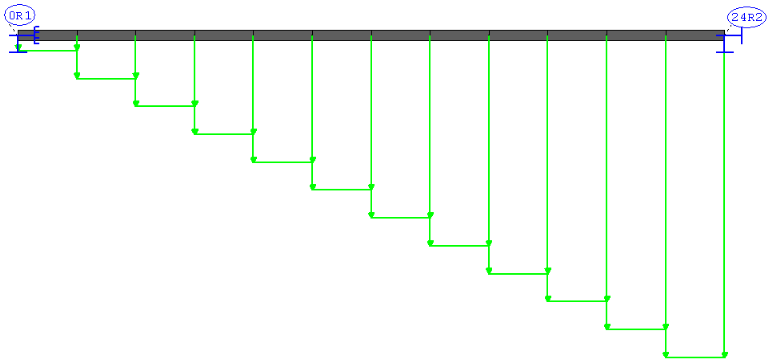Figure 8: 2 inch model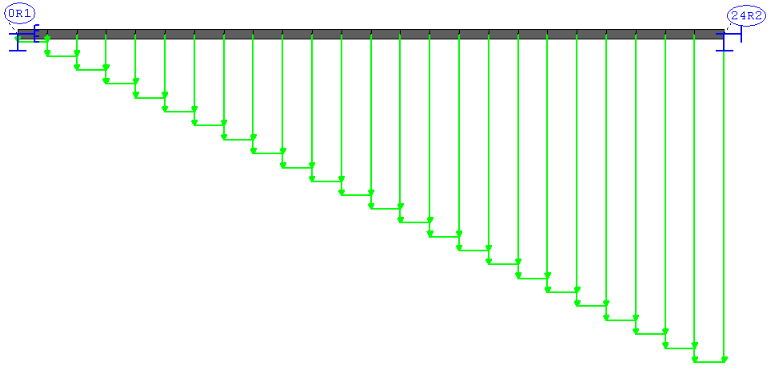Figure 7: 1 inch model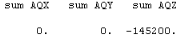Figure 10: W [lbf] from the Lc Dead Weight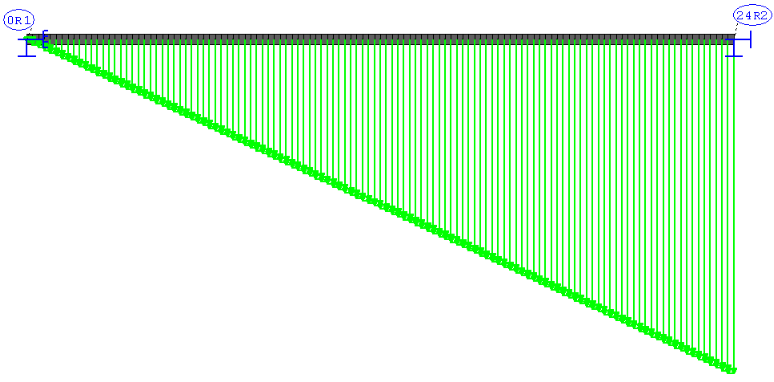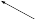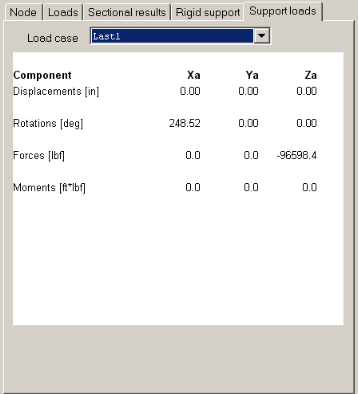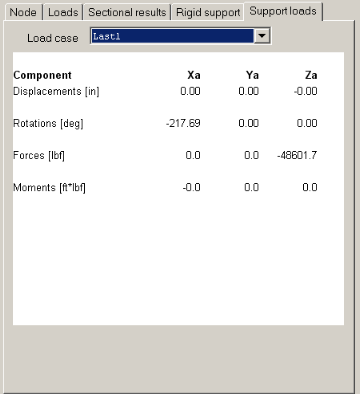Figure 9: 0,2 inch model with the support loads FR1, FR2

## 1.4 Result comparisons

 Version Value [lbf Reference 0 R1 [lbf] Rohr2 0 R1 [lbf] Difference 0 R1 [%] Reference 24 R2 [lbf] Rohr2 24 R2 [lbf] Difference 24 R2 [%]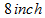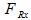48000 51266,7 <6,81 96000 93933,4 <2,3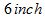50100,0 <4,38 95100,1 <0,95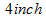49266,7 <2,64 95933,4 <0,07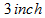48975,0 <2,04 96225,1 <0,24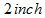48766,7 <1,60 96433,4 <0,46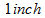48641,7 <1,34 96558,4 <0,59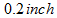48601,7 <1,26 96598,4 <0,63

Table 2: Comparison of the support loads with the reference

 Version Value Reference (Timoshenko) [lbf] Rohr2 [lbf] Difference [%] All seven144000 145200 <0,84

Table 3: Comparison of the sum of support loads

## 1.5 Conclusion

The results become more precise with every version. This accounts for the parts, which become smaller. The results approach to an ideally triangle-line load. One other condition which is given in the reference is, that the support load at the two points 0 R1 and 24 R2 reach ordained values (see table 2and table 3 ). It is to remark, that the dimensions, lengths, loads and materials are free selectable, not given by Timoshenko.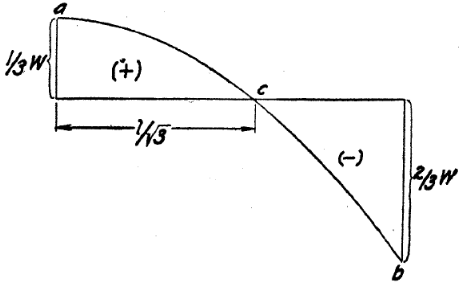Figure 11: force curve by Timoshenko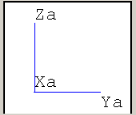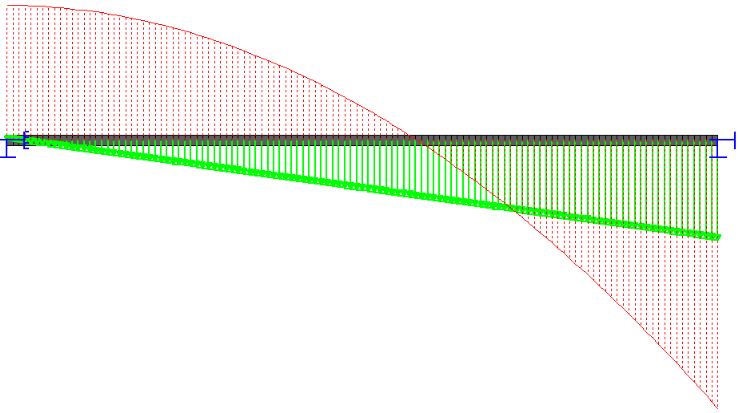Figure 12: force curve of 0,2 inch Model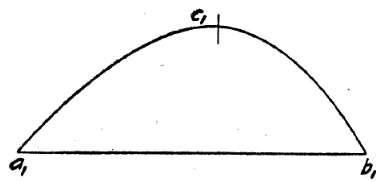Figure 13: moment curve by Timoshenko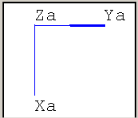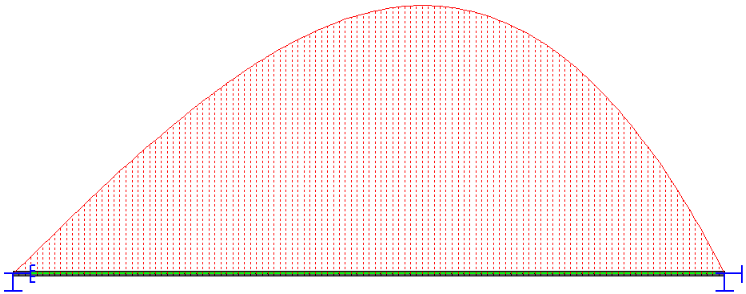Figure 14: moment curve of 0,2 inch Model

The figures also show, that the course of force- and moment-curve is exactly the same as in the reference.

## 1.6 Files

R007_inch.r2w

R007_mm.r2w

R2_stresses_7

SIGMA Ingenieurgesellschaft mbH www.rohr2.com